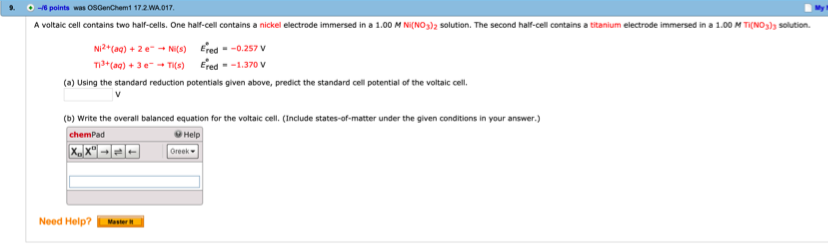# A voltaic cell contains two half-cells. One half-cell contains a nickel electrode immersed in a 1.00 M Ni(NO3)2 solution. The second half-cell contains a titanium electrode immersed in a 1.00 M Ti(NO3)3 solution. Ni2+(aq) + 2 e- -0 Ni(s)Ered0 = -0.257 V Ti3+(aq) + 3 e- Ti(s)Ered0 = -1.370 V (a) Using the standard reduction potentials given above, predict the standard cell potential of the voltaic cell. V (b) Write the overall balanced equation for the voltaic cell. (Include states-of-matter under the given conditions in your answer.)